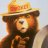# Convert Three-Day Rolling Pivot to ThinkorSwim

L

#### lee-I-O=coke-A

##### New member
Found this in Investopdia. Can someone convert this into thinkscript. Please. It is called "Rolling 3 day pivots"
Here is the calculation for the three-day rolling pivot:

• Three-day rolling pivot price = (three-day high + three-day low + close) / 3
• Second number = (three-day high + three-day low) / 2
• Pivot differential = daily pivot price – second number
• Three-day rolling pivot range high = daily pivot price + pivot differential
• Three-day rolling pivot range low = daily pivot price – pivot differential
The formulas for the high and low piv t range are as follows:

The Pivot= (3day High + 3day Low + Close)/3 The Midpoint= (3day High + 3 day Low)/2

Pivot Range= Absolute Value (Pivot – Midpoint) Pivot High= Pivot + Range

Pivot Low= Pivot – Range

•santamyduudeHow do I convert this ATR% chart study into a scanner watchlist column? Questions 5